#Rule 26: Complex FractionsTo multiply add or subtract two complex fractions, convert the fractions to simple fractions and follow the steps you use to add or subtract two simple fractions.Example:
Calculate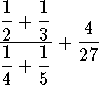.

Convert the numerator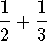of the complex fraction to a simple fraction.can be written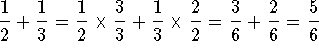. Convert the denominator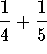of the complex fraction to a simple fraction.can be written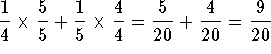.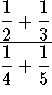can now be written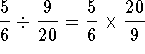.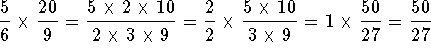. The problem can now be written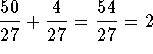.

### If you would like to review another example, click on the word Example. Work the following problems and click on Answer if you want to check the answer and review the solution.

Problem 1:
Simplify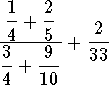Problem 2:
Simplify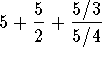.

Problem 3:
Simplify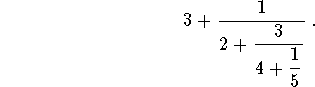[Simple Fractions] [Complex Fractions] [Compound Fractions]
[Decimals] [Percentage] [Rules]

[Algebra] [Trigonometry] [Complex Variables]S.O.S MATHematics home page

Do you need more help? Please post your question on our S.O.S. Mathematics CyberBoard.Author: Nancy Marcus